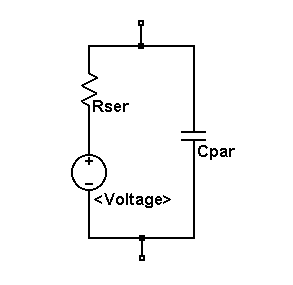# V. Voltage Source

Symbol Names: VOLTAGE, BATTERY

Syntax: Vxxx n+ n- <voltage> [AC=<amplitude>]

+ [Rser=<value>] [Cpar=<value>]

This element sources a constant voltage between nodes n+ and n-. For AC analysis, the value of AC is used as the amplitude of the source at the analysis frequency. A series resistance and parallel capacitance can be defined. The equivalent circuit is:Voltage sources have historically been used as the current meters in SPICE and are used as current sensors for current-controlled elements. If Rser is specified, the voltage source can not be used as a sense element for F, H, or W elements. However, the current of any circuit element, including the voltage source, can be plotted.

Syntax: Vxxx n+ n- PULSE(V1 V2 Tdelay Trise Tfall Ton Tperiod Ncycles )

Time-dependent pulsed voltage source

 Name Description Units Voff Initial value V Von Pulsed value V Tdelay Delay sec Tr Rise time sec Tf Fall time sec Ton On time sec Tperiod Period sec Ncycles Number of cycles(Omit for free-running pulse function) cycles

Syntax: Vxxx n+ n- SINE(Voffset Vamp Freq Td Theta Phi Ncycles)

Time-dependent sine wave voltage source.

 Name Description Units Voffset DC offset V Vamp Amplitude V Freq Frequency Hz Td Delay sec Theta Damping factor 1/sec Phi Phase of sine wave degrees Ncycles Number of cycles(Omit for free-running pulse function) cycles

For times less than Td or times after completing Ncycles, have run, the output voltage is given by

Voffset+Vamp*sin(pi*Phi/180)

Otherwise the voltage is given by

Voffset+Vamp*exp(-(time-Td)*Theta)*sin(2*pi*Freq*(time-Td)+pi*Phi/180)

The damping factor, Theta, is the reciprocal of the decay time constant.

Syntax: Vxxx n+ n- EXP(V1 V2 Td1 Tau1 Td2 Tau2)

Time-dependent exponential voltage source

 Name Description Units V1 Initial value V V2 Pulsed value V Td1 Rise delay time sec Tau1 Rise-time constant sec Td2 Fall delay time sec Tau2 Fall-time constant sec

For times less than Td1, the output voltage is V1. For times between Td1 and Td2 the voltage is given by

V1+(V2-V1)*(1-exp(-(time-Td1)/Tau1)).

For times after Td2 the voltage is given by

V1+(V2-V1)*(1-exp(-(time-Td1)/Tau1))

+(V1-V2)*(1-exp(-(time-Td2)/Tau2)).

Syntax: Vxxx n+ n- SFFM(Voff Vamp Fcar MDI Fsig)

Time-dependent single frequency FM voltage source.

 Name Description Units Voff DC offset V Vamp Amplitude V Fcar Carrier frequency Hz MDI Modulation index - Fsig Signal frequency Hz

The voltage is given by

Voff+Vamp*sin((2.*pi*Fcar*time)+MDI*sin(2.*pi*Fsig*time)).

Syntax: Vxxx n+ n- PWL(t1 v1 t2 v2 t3 v3...)

Arbitrary Piece-wise linear voltage source.

For times before t1, the voltage is v1. For times between t1 and t2, the voltage varies linearly between v1 and v2. There can be any number of time, voltage points given. For times after the last time, the voltage is the last voltage.

Syntax: Vxxx n+ n- wavefile=<filename> [chan=<nnn>]

This allows a .wav file to be used as an input to LTspice. <filename> is either a full, absolute path for the .wav file or a relative path computed from the directory containing the simulation schematic or netlist.  Double quotes may be used to specify a path containing spaces.  The .wav file may contain up to 65536 channels, numbered 0 to 65535.  Chan may be set to specify which channel is used.  By default, the first channel, number 0, is used.  The .wav file is interpreted as having a full scale range from -1V to 1V.

This source only has meaning in a .tran analysis.